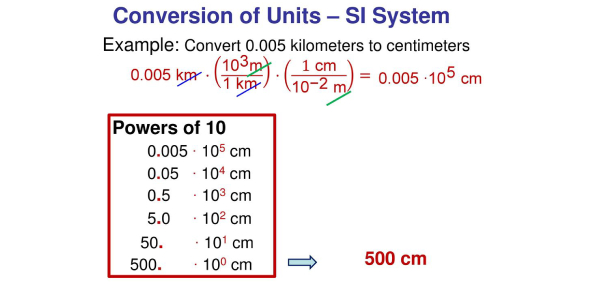# Unit Conversion Quiz Questions And Answers

Reviewed by Janaisa Harris
Janaisa Harris, BA-Mathematics |
Mathematics Expert
Review Board Member
Ms. Janaisa Harris, an experienced educator, has devoted 4 years to teaching high school math and 6 years to tutoring. She is now broadening her educational impact by engaging in curriculum mapping for her county. This endeavor enriches her understanding of educational strategies and their implementation.
, BA-Mathematics
Approved & Edited by ProProfs Editorial Team
The editorial team at ProProfs Quizzes consists of a select group of subject experts, trivia writers, and quiz masters who have authored over 10,000 quizzes taken by more than 100 million users. This team includes our in-house seasoned quiz moderators and subject matter experts. Our editorial experts, spread across the world, are rigorously trained using our comprehensive guidelines to ensure that you receive the highest quality quizzes.
| By MsCyr
M
MsCyr
Community Contributor
Quizzes Created: 2 | Total Attempts: 15,054
Questions: 10 | Attempts: 15,057SettingsAre you ready for a unit conversion quiz? The conversion of units is the conversion between different units of measurement for the same quantity. Typically, through multiplicative conversion factors. It is used to modify the units of a precise quantity without changing their value. The method of conversion is contingent upon the specific situation and the intended purpose. If you want to learn more about unit conversion, this is the quiz for you.

• 1.

### How many inches are in 45.6 cm? (There are 2.54cm in 1 inch)

• A.

18.0 inches

• B.

9 inches

• C.

17.952 inches

• D.

115.8 inches

A. 18.0 inches
Explanation
45.6cm x 1in/2.54cm = 17.952 inches BUT only 3 sigfigs, so 18.0

Rate this question:

• 2.

### How many centimeters are in 1.23 x 10^-6 kilometers?

• A.

0.123 cm

• B.

0.1234 cm

• C.

0.12 cm

• D.

0.13 cm

A. 0.123 cm
Explanation
0.00000123km x 1000m/1km x 100cm/1m = 0.123 3sigfigs

Rate this question:

• 3.

### Approximately how many hours are there in 34.5 years?

• A.

302,000 hrs

• B.

302,220 hrs

• C.

0.00394 hrs

• D.

0.00393835 hrs

B. 302,220 hrs
Explanation
To calculate the number of hours in 34.5 years, you can use the following calculation:

Number of hours in a year × Number of years

There are typically 365 days in a year, and each day has 24 hours. So, in a non-leap year:

365 days/year × 24 hours/day = 8,760 hours/year

Now, you can multiply this by the number of years:

8,760 hours/year × 34.5 years = 302,220 hours

So, there are approximately 302,220 hours in 34.5 years.

Rate this question:

• 4.

### How many inches are there in 355 millimeters? (25.4 mm in an inch)

• A.

14.0 inches

• B.

13.90 inches

• C.

140.0 inches

• D.

139.0 inches

A. 14.0 inches
Explanation
355mm x 1cm/10mm x 1in/2.54cm = 13.976 BUT 3 sigfigs or 355mm x 1in/25.4mm = 13.976

Rate this question:

• 5.

### 10 meters = ______ inches.

• A.

278

• B.

393

• C.

403

• D.

782

B. 393
Explanation
10 meters is equal to 1000 centimeters. Since there are 2.54 centimeters in an inch, we can convert 1000 centimeters to inches by dividing by 2.54. The result is approximately 393 inches.

Rate this question:

• 6.

### 2.1 meter is equal to

• A.

4 feet

• B.

5 feet

• C.

7 feet

• D.

10 feet

C. 7 feet
Explanation
2.1 meters is equal to 6.89 feet. Since none of the options given match this value exactly, the correct answer is not available.

Rate this question:

• 7.

### Convert 14 mm to its equivalent measurement in m.

• A.

0.014m

• B.

0.0014m

• C.

0.14m

• D.

1400

A. 0.014m
Explanation
14mm x 1m/1000mm

Rate this question:

• 8.

### Which of the following is a TRUE metric conversion factor?

• A.

1mm = 1000m

• B.

1000mm = 1m

• C.

100m = 1cm

• D.

1m = 1000 km

B. 1000mm = 1m
Explanation
The given statement "1000mm = 1m" is a true metric conversion factor because it represents the relationship between millimeters and meters. It states that there are 1000 millimeters in 1 meter, which is a standard conversion factor in the metric system.

Rate this question:

• 9.

### Which of the following is a FALSE metric conversion factor?

• A.

100cm = 1m

• B.

1000g = 1 kg

• C.

1g = 1000mg

• D.

1000g = 1g

D. 1000g = 1g
Explanation
Think:
Balanced Scale

Rate this question:

• 10.

### Convert 57mL to its equivalent measurement in L.

• A.

0.057 L

• B.

57000 L

• C.

0.0057 L

• D.

5700 L

A. 0.057 L
Explanation
57 mL x 1L/ 1000mL = L

Rate this question:

Janaisa Harris |BA-Mathematics |
Mathematics Expert
Ms. Janaisa Harris, an experienced educator, has devoted 4 years to teaching high school math and 6 years to tutoring. She is now broadening her educational impact by engaging in curriculum mapping for her county. This endeavor enriches her understanding of educational strategies and their implementation.

Related TopicsBack to top